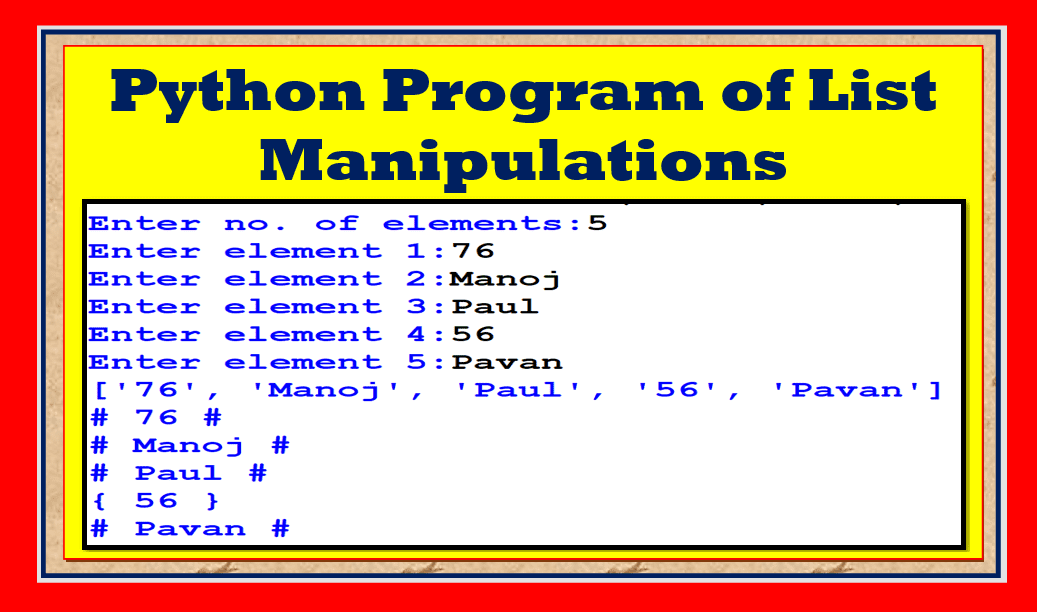In this article, python program list manipulations computer science class 11 I will provide programs based on list manipulations class 11 computer science. Let us begin!

Topics Covered

## python program list manipulations computer science class 11

Let us start it with the following:

Write a program in python to enclose the elements of list with {}, if it is a number and enclose the elements with # if it is not a number.

The output should be :

Enter no. of elements:5
Enter element 1:76
Enter element 2:Manoj
Enter element 3:Paul
Enter element 4:56

Enter element 5:Pavan
[’76’, ‘Manoj’, ‘Paul’, ’56’,’Pavan’]
{ 76 }

#Paul#

{56}

#Pavan#

## Steps

1. Accept the list elements
2. Traverse the list elements using for loop
3. Use isdigit() method to check the digits used in list elements and to enclose them with {}
4. in else enclose the remaining text with #

## Python code

See the code python program list manipulations computer science class 11.

Solution 1:

``````l=eval(input("Enter list:"))
print(l)
for i in l:
if str(i).isdigit():
print('{',i,'}')
else:
print('#',i,'#')``````

Steps:

1. Declare an empty list
2. Take a new variable to prompt no. of elements
3. Use for loop to accept n no. of elements
4. Accept the values to add into the list
5. Append the values to the list
6. Traverse the list
7. use if condition to enclose integers with {} and convert the digits into integers
8. Use if condition to check the type integer with {}
9. Write the code to enclose the text with #

See the code for python program list manipulations computer science class 11.

Solution 2:

``````l=[]
n=int(input("Enter no. of elements:"))
for i in range(n):
v=input('Enter element '+str(i+1)+':')
l.append(v)
print(l)

for i in l:
if i in '1234567890':
i=int(i)
if type(i)==int:
print('{',i,'}')
else:
print('#',i,'#')``````